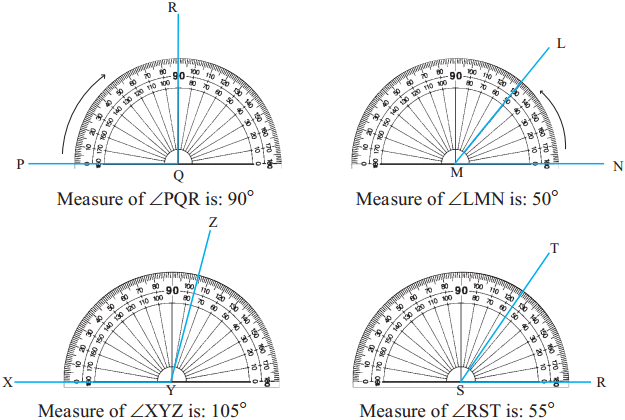# Maharashtra Board Class 5 Maths Solutions Chapter 6 Angles Problem Set 24

Balbharti Maharashtra Board Class 5 Maths Solutions Chapter 6 Angles Problem Set 24 Textbook Exercise Important Questions and Answers.

## Maharashtra State Board Class 5 Maths Solutions Chapter 6 Angles Problem Set 24

Complete the following table.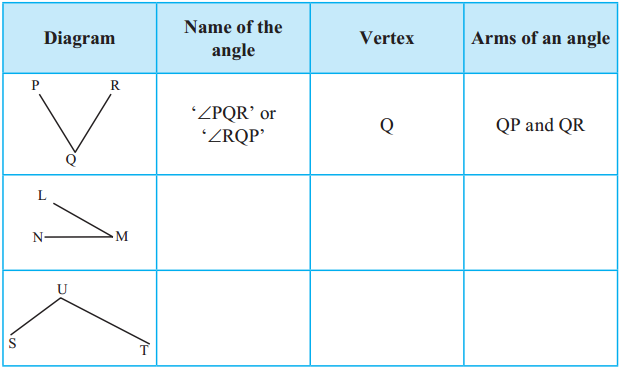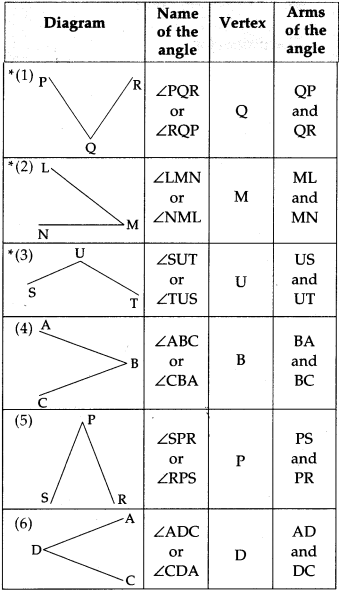The protractor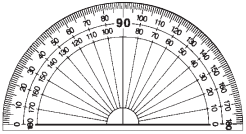A protractor is used to measure an angle and also to draw an angle according to a given measure.

The picture opposite shows a protractor.

A protractor is semi-circular in shape.

The semi-circular edge of a protractor is divided into 180 equal parts. Each part is ‘one degree’. ‘One degree’ is written as ‘1°’.

The divisions on a protractor, i.e., the degrees can be marked in two ways. The divisions 0, 10, 20, 30,…180 are marked anticlockwise or from right to left; the divisions 0, 10, 20, 30,…180 are also marked clockwise, or serially from left to right.

The centre of the circle of which the protractor is a half part, is called the centre of the protractor. A diameter of that circle is the baseline or line of reference of the protractor.

Measuring angles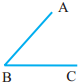Observe how to measure ∠ABC given alongside, using a protractor.

1. First, put the centre of the protractor on the vertex B of the angle. Place the baseline of the protractor exactly on arm BC. The arms of the angle do not reach the divisions on the protractor.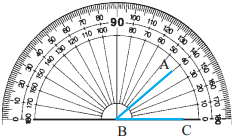2. At such times, set the protractor aside and extend the arms of the angle. Extending the arms of the angle does not change the measure of the angle.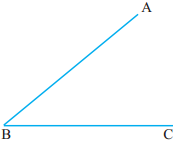3. The angle is measured starting from the zero on that side of the vertex on which the arm of the angle lies. Here, the arm BC is on the right of the vertex B. Therefore, count the divisions starting from the 0 on the right. See which mark falls on arm BA. Read the number on that mark. This number is the measure of the angle.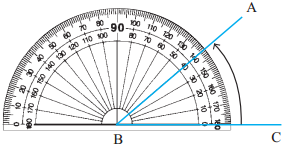The measure of∠ABC is 40°.

We can measure the same ∠ABC by positioning the protractor differently.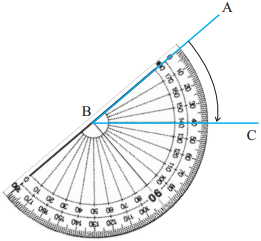1. First put the centre point of the protractor on vertex B of the angle. Align the baseline of the protractor with arm BA.
2. Find the 0 mark on the side of BA. Count the marks starting from the 0 on the side of point A. See which mark falls on arm BC. Read the number at that point.

Observe that here, too, the measure of ∠ABC is 40°.

See how the angles given below have been measured with the help of a protractor.